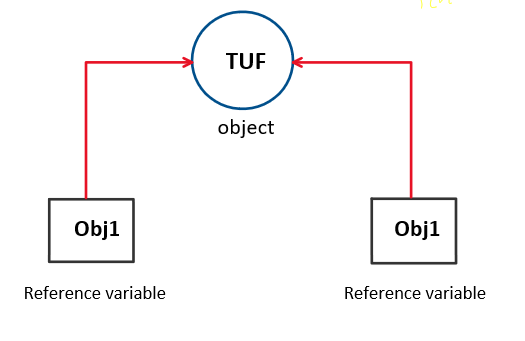# Java classes and objects

### Introduction:

Java is an object-oriented programming language that uses classes and objects to store and execute data using data members and member functions.

Let’s try understanding what java class is.

### Java Class

Class is a user-defined data type consisting of data members and member functions used to store and process the data. Classes work as the root branches of OOP giving rise to various programming paradigms like encapsulation, inheritance, polymorphism, etc.

A simple class in java looks like this

```class TUF {
int ans; // fields/ instance variables
public void printAns() { // member function/ methods

}
}
```

The variables defined in the class are instance variables or fields and these combine with the member functions/methods to form members of the class. Once we create a class, no memory is allocated until and unless an instance of that class is created i.e. an object is created. Now let’s try creating a real existence of this class which can be done by creating an object of this class.

### Java Objects

In other data types, we declare a variable of that data type. For example,

```int a;
int b;
```

The same goes with classes and objects too. Only the terminology changes.

Here we use the class name as the data type and the reference variable is known as the object.

`TUF obj=new TUF();`

Here TUF is the class name(data type) and obj is the object i.e. reference variable. Once we run this statement, a real-world existence of the class TUF would be created.

We can try creating multiple objects of the same class i.e. multiple instances of the same class

```TUF obj1=new TUF();
TUF obj2=new TUF();
```

Each object will create its own instance with a separate copy of all instance variables and methods within the object.

We can use multiple reference variables for a single object. For example,

```TUF obj1=new TUF();
obj1=obj2;
```

Here both obj1 and obj2 would be referring to the same object, thus no duplicate object is created and no extra memory is allocated. The following diagram explains this statement.### Accessing members of class using objects

Let’s define a class name TUF,

```class TUF {
int value;
public void printValue() {
System.out.println("Value is " + value);
}
}
```

Now let’s create an object for this class.

`TUF obj1=new TUF();`

To use the members of the class with the help of objects, one can use the “.” (dot) operator.

The following code explains the above approach

```obj1.Value=30;
ob1.printValue;
```

The scope of classes and objects is not limited to this article. Once you get a clear understanding of how classes work and how memory management is done, you can apply other paradigms which can be useful in building safe and fool-proof applications using JAVA.

Special thanks to Yash Mishra for contributing to this article on takeUforward. If you also wish to share your knowledge with the takeUforward fam, please check out this article